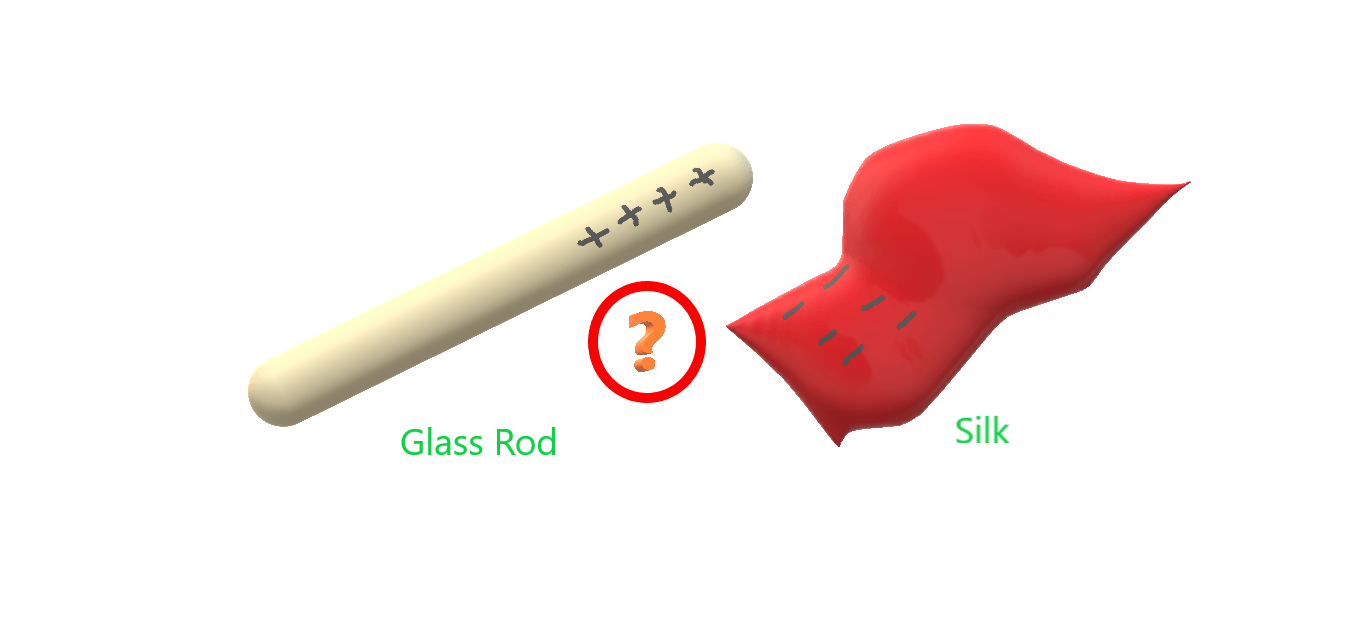#### Why always glass rod got positive charge on rubbing with silk

You should have a confusion in your mind , if you have one. Far more than 2600 years when we rub a glass rod with silk it gets charged positively over and again, not even a single time it is negatively charged. If something is following in some manner for such a long duration, then we are forced to think that there is some genuine reason, some law governing the phenomenon. Then think what happens with glass? Why always electrons flow from glass to silk.

I can give the answer in one line – By saying that this due to work function and quit it. But then question arises what is work function? Let us try to understand what is work function. Every material is made of molecules and every molecule is made of atoms. And finally we know the structure of atom. every atom consists of different number of electrons, protons and neutrons, giving different strength to electrical forces between electrons and nucleus. Hence if wanna remove some electron from one atom, we require different amount of energy for different atoms. Leave it for now. Lets do one experiment.

Take a thread. Apply little force and then gradually increase the force. see when the thread breaks – not the process. You gradually increased the force and at certain value of force thread breaks, and not at a lower value. What will happen if you apply a force greater than this force….Are you sure? Very good, the thread will break. Now we can conclude that if we apply a force less than breaking force ( I named it ), the thread will not break. But if we apply a force equal to or greater than , then the thread will break.

This gives the concept of minimum force required for breaking the thread, which can be called as breaking force.

Similarly, the minimum force required to remove an electron from atom is called its work function.